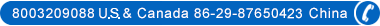A reputable China tour operator & China travel agency from 1987# Temperature Conversion

Chinese people use Celsius more than Fahrenheit. Here foreign travelers on China tours can use the following formulas to convert the temperature between Fahrenheit to Celsius.

Temperature Conversion Formulas

 From Fahrenheit (F) to Celsius (C) From Celsius (C) to Fahrenheit (F) 1. Start with (F) 1. Start with (C) 2. Subtract 32 2. Multiply by 9 3. Multiply by 5 3. Divide by 5 4. Divide by 9 4. Add on 32 5. The answer is (C) 5. The answer is (F)

Want a quick temperature conversion:

Fahrenheit: 'F (Fahrenheit)= ℃ X 9/5+32

Celsius: ℃ (Celsius or Centigrade)= 5/9X('F-32)

### China Travel Guide

Tel: 8003209088 (US & Canada) 86-29-87650440 (China local number) Email: info@china-tour.cn